Download Presentation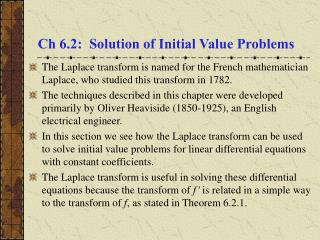Ch 6.2: Solution of Initial Value Problems

# Ch 6.2: Solution of Initial Value Problems - PowerPoint PPT Presentation

Ch 6.2: Solution of Initial Value Problems . The Laplace transform is named for the French mathematician Laplace, who studied this transform in 1782. The techniques described in this chapter were developed primarily by Oliver Heaviside (1850-1925), an English electrical engineer.I am the owner, or an agent authorized to act on behalf of the owner, of the copyrighted work described.
Download Presentation## Ch 6.2: Solution of Initial Value Problems

An Image/Link below is provided (as is) to download presentation

Download Policy: Content on the Website is provided to you AS IS for your information and personal use and may not be sold / licensed / shared on other websites without getting consent from its author.While downloading, if for some reason you are not able to download a presentation, the publisher may have deleted the file from their server.

- - - - - - - - - - - - - - - - - - - - - - - - - - E N D - - - - - - - - - - - - - - - - - - - - - - - - - -
Presentation Transcript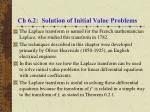Ch 6.2: Solution of Initial Value Problems
• The Laplace transform is named for the French mathematician Laplace, who studied this transform in 1782.
• The techniques described in this chapter were developed primarily by Oliver Heaviside (1850-1925), an English electrical engineer.
• In this section we see how the Laplace transform can be used to solve initial value problems for linear differential equations with constant coefficients.
• The Laplace transform is useful in solving these differential equations because the transform of f' is related in a simple way to the transform of f, as stated in Theorem 6.2.1.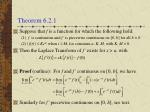Theorem 6.2.1
• Suppose that f is a function for which the following hold:

(1) f is continuous and f' is piecewise continuous on [0, b] for all b > 0.

(2) | f(t) | Keat when t M, for constants a, K, M,with K, M > 0.

• Then the Laplace Transform of f' exists for s > a, with
• Proof (outline): For f and f' continuous on [0, b], we have
• Similarly for f' piecewise continuous on [0, b], see text.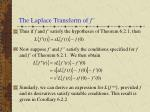The Laplace Transform of f'
• Thus if f and f' satisfy the hypotheses of Theorem 6.2.1, then
• Now suppose f' and f '' satisfy the conditions specified for f and f' of Theorem 6.2.1. We then obtain
• Similarly, we can derive an expression for L{f(n)}, provided f and its derivatives satisfy suitable conditions. This result is given in Corollary 6.2.2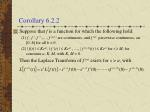Corollary 6.2.2
• Suppose that f is a function for which the following hold:

(1) f , f ', f '' ,…, f(n-1) are continuous, and f(n) piecewise continuous, on [0, b] for all b > 0.

(2) | f(t) | Keat, | f '(t) | Keat , …, | f (n-1)(t) | Keat for t M, for constants a, K, M,with K, M > 0.

Then the Laplace Transform of f(n) exists for s > a, with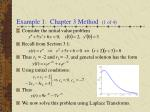Example 1: Chapter 3 Method (1 of 4)
• Consider the initial value problem
• Recall from Section 3.1:
• Thus r1 = -2 and r2 = -3, and general solution has the form
• Using initial conditions:
• Thus
• We now solve this problem using Laplace Transforms.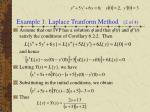Example 1: Laplace Tranform Method (2 of 4)
• Assume that our IVP has a solution  and that '(t) and ''(t) satisfy the conditions of Corollary 6.2.2. Then

and hence

• Letting Y(s) = L{y}, we have
• Substituting in the initial conditions, we obtain
• Thus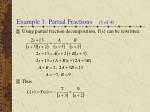Example 1: Partial Fractions (3 of 4)
• Using partial fraction decomposition, Y(s) can be rewritten:
• Thus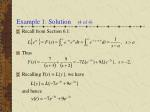Example 1: Solution (4 of 4)
• Recall from Section 6.1:
• Thus
• Recalling Y(s) = L{y}, we have

and hence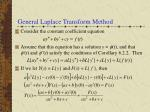General Laplace Transform Method
• Consider the constant coefficient equation
• Assume that this equation has a solution y = (t), and that '(t) and ''(t) satisfy the conditions of Corollary 6.2.2. Then
• If we let Y(s) = L{y} and F(s) = L{ f }, then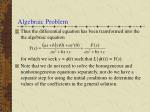Algebraic Problem
• Thus the differential equation has been transformed into the the algebraic equation

for which we seek y = (t) such that L{(t)} = Y(s).

• Note that we do not need to solve the homogeneous and nonhomogeneous equations separately, nor do we have a separate step for using the initial conditions to determine the values of the coefficients in the general solution.Characteristic Polynomial
• Using the Laplace transform, our initial value problem

becomes

• The polynomial in the denominator is the characteristic polynomial associated with the differential equation.
• The partial fraction expansion of Y(s) used to determine  requires us to find the roots of the characteristic equation.
• For higher order equations, this may be difficult, especially if the roots are irrational or complex.Inverse Problem
• The main difficulty in using the Laplace transform method is determining the functiony = (t) such that L{(t)} = Y(s).
• This is an inverse problem, in which we try to find  such that (t) = L-1{Y(s)}.
• There is a general formula for L-1, but it requires knowledge of the theory of functions of a complex variable, and we do not consider it here.
• It can be shown that if f is continuous with L{f(t)} = F(s), then f is the unique continuous function with f(t) = L-1{F(s)}.
• Table 6.2.1 in the text lists many of the functions and their transforms that are encountered in this chapter.Linearity of the Inverse Transform
• Frequently a Laplace transform F(s) can be expressed as
• Let
• Then the function

has the Laplace transform F(s), since L is linear.

• By the uniqueness result of the previous slide, no other continuous function f has the same transform F(s).
• Thus L-1 is a linear operator withExample 2
• Find the inverse Laplace Transform of the given function.
• To find y(t) such that y(t) = L-1{Y(s)}, we first rewrite Y(s):
• Using Table 6.2.1,
• ThusExample 3
• Find the inverse Laplace Transform of the given function.
• To find y(t) such that y(t) = L-1{Y(s)}, we first rewrite Y(s):
• Using Table 6.2.1,
• ThusExample 4
• Find the inverse Laplace Transform of the given function.
• To find y(t) such that y(t) = L-1{Y(s)}, we first rewrite Y(s):
• Using Table 6.2.1,
• ThusExample 5
• Find the inverse Laplace Transform of the given function.
• To find y(t) such that y(t) = L-1{Y(s)}, we first rewrite Y(s):
• Using Table 6.2.1,
• ThusExample 6
• Find the inverse Laplace Transform of the given function.
• To find y(t) such that y(t) = L-1{Y(s)}, we first rewrite Y(s):
• Using Table 6.2.1,
• ThusExample 7
• Find the inverse Laplace Transform of the given function.
• To find y(t) such that y(t) = L-1{Y(s)}, we first rewrite Y(s):
• Using Table 6.2.1,
• ThusExample 8
• Find the inverse Laplace Transform of the given function.
• To find y(t) such that y(t) = L-1{Y(s)}, we first rewrite Y(s):
• Using Table 6.2.1,
• ThusExample 9
• For the function Y(s) below, we find y(t) = L-1{Y(s)} by using a partial fraction expansion, as follows.Example 10
• For the function Y(s) below, we find y(t) = L-1{Y(s)} by completing the square in the denominator and rearranging the numerator, as follows.
• Using Table 6.1, we obtainExample 11: Initial Value Problem (1 of 2)
• Consider the initial value problem
• Taking the Laplace transform of the differential equation, and assuming the conditions of Corollary 6.2.2 are met, we have
• Letting Y(s) = L{y}, we have
• Substituting in the initial conditions, we obtain
• ThusExample 11: Solution (2 of 2)

• Completing the square, we obtain
• Thus
• Using Table 6.2.1, we have
• Therefore our solution to the initial value problem isExample 12: Nonhomogeneous Problem (1 of 2)
• Consider the initial value problem
• Taking the Laplace transform of the differential equation, and assuming the conditions of Corollary 6.2.2 are met, we have
• Letting Y(s) = L{y}, we have
• Substituting in the initial conditions, we obtain
• ThusExample 12: Solution (2 of 2)

• Using partial fractions,
• Then
• Solving, we obtain A = 2, B = 5/3, C = 0, and D = -2/3. Thus
• Hence